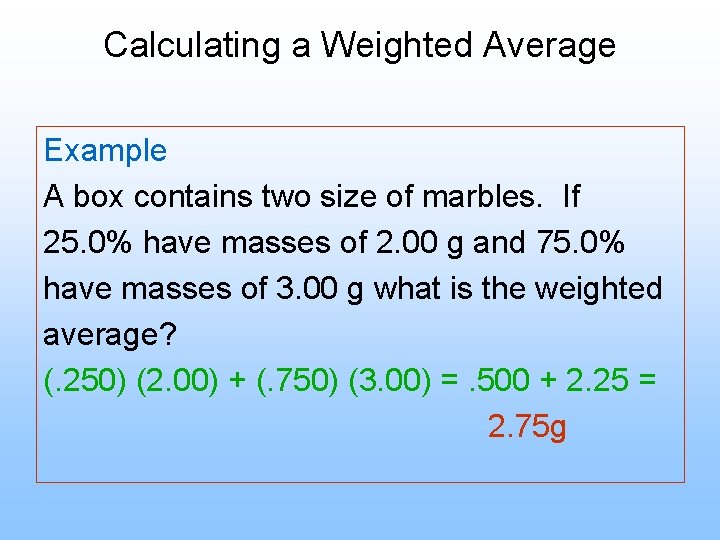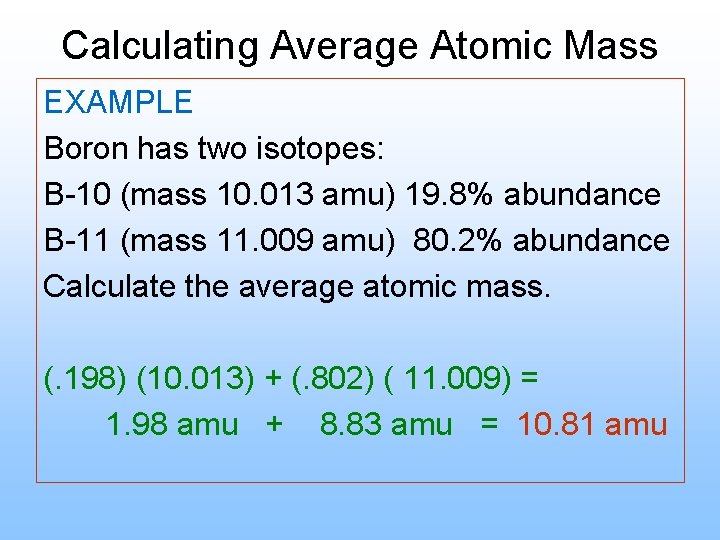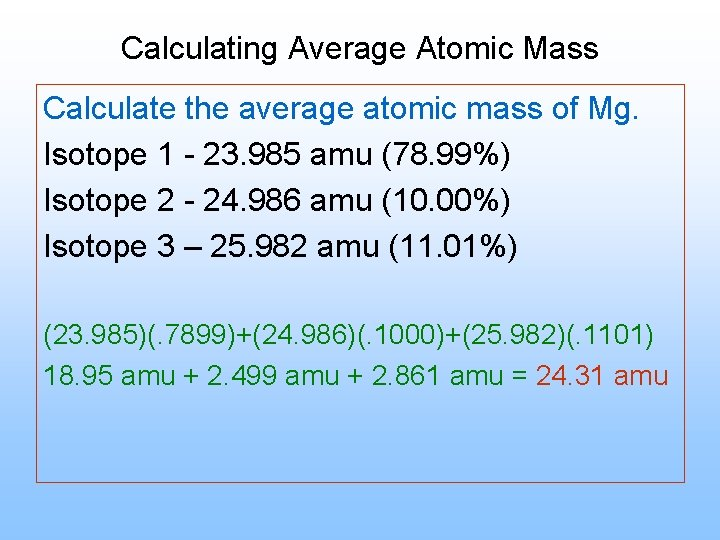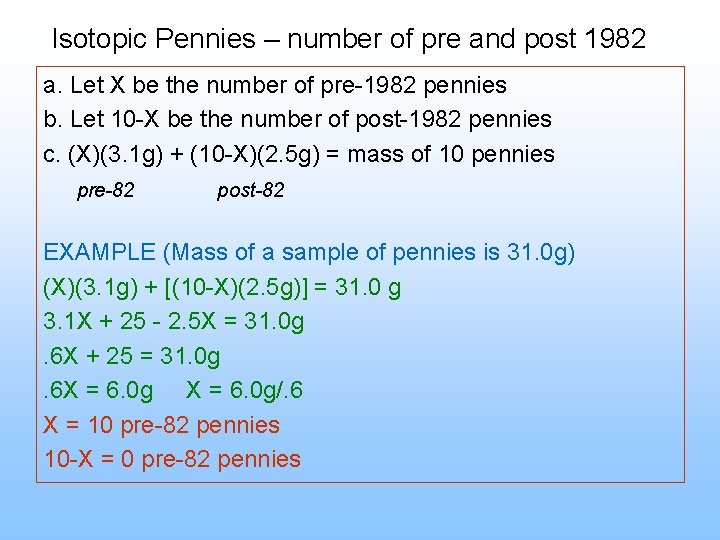# Average Atomic Mass What is average atomic mass

• Slides: 7Average Atomic Mass What is average atomic mass? Average atomic mass is the weighted average of the atomic masses of the naturally occurring isotopes of an elementCalculating a Weighted Average Example A box contains two size of marbles. If 25. 0% have masses of 2. 00 g and 75. 0% have masses of 3. 00 g what is the weighted average? (. 250) (2. 00) + (. 750) (3. 00) =. 500 + 2. 25 = 2. 75 gCalculating Average Atomic Mass EXAMPLE Boron has two isotopes: B-10 (mass 10. 013 amu) 19. 8% abundance B-11 (mass 11. 009 amu) 80. 2% abundance Calculate the average atomic mass. (. 198) (10. 013) + (. 802) ( 11. 009) = 1. 98 amu + 8. 83 amu = 10. 81 amuCalculating Average Atomic Mass Calculate the average atomic mass of Mg. Isotope 1 - 23. 985 amu (78. 99%) Isotope 2 - 24. 986 amu (10. 00%) Isotope 3 – 25. 982 amu (11. 01%) (23. 985)(. 7899)+(24. 986)(. 1000)+(25. 982)(. 1101) 18. 95 amu + 2. 499 amu + 2. 861 amu = 24. 31 amuAverage Atomic Mass Helium has two naturally occurring isotopes, He-3 and He-4. The atomic mass of helium is 4. 003 amu. Which isotope is more abundant in nature? He-4 is more abundant in nature because the atomic mass is closer to the mass of He-4 than to the mass of He-3.Isotopic Pennies – number of pre and post 1982 a. Let X be the number of pre-1982 pennies b. Let 10 -X be the number of post-1982 pennies c. (X)(3. 1 g) + (10 -X)(2. 5 g) = mass of 10 pennies pre-82 post-82 EXAMPLE (Mass of a sample of pennies is 31. 0 g) (X)(3. 1 g) + [(10 -X)(2. 5 g)] = 31. 0 g 3. 1 X + 25 - 2. 5 X = 31. 0 g. 6 X + 25 = 31. 0 g. 6 X = 6. 0 g/. 6 X = 10 pre-82 pennies 10 -X = 0 pre-82 penniesIsotopic Penny Lab- Average Atomic Mass Calculate percent of pre-82 and post-82 pennies # of pre-82 pennies x 100% 10 # post-82 pennies x 100% 10 Calculate the average atomic mass of coinium (% pre-82)(3. 1 g) + (% post-82)(2. 5) = average atomic mass